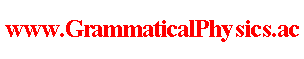since 2006 Help　Sitemap < Forum > < Problems > < Quantum History Theory > < Examine Probability Formula > Φ[χ] = Σj exp[α∫dt φj(χ(t), t)], [dar(f; a, a + 1/α)]pre Φ[χ] = ∫dx f(x)* Φ[ξ(□, x)] = ∫dx f(x)* Σj exp[α∫dt φj(ξ(t, x), t)] = Σj ∫dx f(x)* exp[α∫aa+1/α dt φj(x, t)] exp[α∫t'＜a or t'≧a+1/α dt' φj(χ(t'), t')] dar(f; a, a + 1/α) Φ[χ(a,a+1/α)] = [dar(f; a, a + 1/α)]pre Φ[χ] = Σj ∫dx f(x)* exp[α∫aa+1/α dt φj(x, t)] exp[α∫t'＜a or t'≧a+1/α dt' φj(χ(t'), t')] = Σj ∫dx f(x)* exp[α∫aa+1/α dt φj(x, t)] exp[α∫-∞a dt' φj(χ(a,a+1/α)(t'), t') + α∫a∞ dt' φj(χ(a,a+1/α)(t'), t'+1/α)]≒ Σj ∫dx f(x)* exp φj(x, a)　　　exp[ Σk＜0 φj(χ(a,a+1/α)(a + k/α), a + k/α) + Σk≧0 φj(χ(a,a+1/α)(a + k/α), a + (k + 1)/α)] ∵ α ≫ 1 dar(f; a, a + 1/α) Φ[χ]≒ Σj ∫dx f(x)* exp φj(x, a) exp[ Σk＜0 φj(χ(a + k/α), a + k/α) + Σk≧0 φj(χ(a + k/α), a + (k + 1)/α)] Let exp φ'j be a solution of the old Schrödinger equation for each j.Let {exp φ'1(□, t), exp φ'2(□, t), ・・・} be an orthonormal basis of the state space of the old quantum mechanics for each t.Let exp φj be a wave function given from exp φ'j by the twist remove normalization for each j. ∫Dχ dar(g; b, b + 1/α) Φ[χ]・{dar(f; a, a + 1/α) Φ[χ]}*≒ Σj,j' [Πk∫dχ(a+k/α)]　　∫dx' g(x')* exp φj'(x', b) exp[ Σk'＜0 φj'(χ(b + k'/α), b + k'/α) + Σk'≧0 φj'(χ(b + k'/α), b + (k' + 1)/α)]　　{∫dx f(x)* exp φj(x, a) exp[ Σk＜0 φj(χ(a + k/α), a + k/α) + Σk≧0 φj(χ(a + k/α), a + (k + 1)/α)]}* = Σj,j' ∫dx' g(x')* exp φj'(x', b) ∫dx f(x) [exp φj(x, a)]*　　Πk＜0 ∫dχ(a+k/α) [exp φj(χ(a + k/α), a + k/α)]* [exp φj(χ(a + k/α), a + k/α)]　　Π0≦k＜α(b-a) ∫dχ(a+k/α) [exp φj(χ(a + k/α), a + (k + 1)/α)]* [exp φj(χ(a + k/α), a + k/α)]　　Πk≧α(b-a) ∫dχ(a+k/α) [exp φj(χ(a + k/α), a + k/α)]* [exp φj(χ(a + k/α), a + k/α)] = Σj,j' ∫dx' g(x')* exp φj'(x', b) ∫dx f(x) [exp φj(x, a)]*　　(δj,j')∞ Π0≦k＜α(b-a) ∫dχ(a+k/α) [exp φj(χ(a + k/α), a + (k + 1)/α)]* [exp φj(χ(a + k/α), a + k/α)] = Σj ＜g|j,b＞＜j,a|f＞ Π0≦k＜α(b-a) ＜j,a+(k+1)/α|j,a+k/α＞≒ Σj ＜g|j,b＞＜j,a|f＞ ∵ ※ = Σj ＜g|U(b,a)|j,a＞＜j,a|f＞= ＜g|U(b,a)|f＞if α(b - a) ∈ N. ---※---Π0≦k＜α(b-a) ∫dχ(a+k/α) [exp φj(χ(a + k/α), a + (k + 1)/α)]* [exp φj(χ(a + k/α), a + k/α)] = Π0≦k＜α(b-a) ＜j,a+(k+1)/α|j,a+k/α＞≒ Π0≦k＜α(b-a) ＜j,a+k/α|j,a+k/α＞ ∵the twist remove normalization = ＜j,a|j,a＞α(b-a) = 1--- However, notice that the new grammar version of the Schrödinger equation has no solution such that Φ[χ] = Σj exp[α∫dt φj(χ(t), t)]. A few approximations caused by the finiteness of α shift the result from the one in the old quantum mechanics.Such an approximation is not needed if α is infinity.I don't think that it is a prediction of the new theory distinct from the old quantum mechanics.I think that it should be understood as the fact that a quantum history and a measurement are less related to each other than in the old quantum mechanics. A prediction of the new theory distinct from the old quantum mechanics will be caused by the entanglement of a quantum history in a time-like direction. --- This article is a rewrite of the article 'Examine Probability Formula' in the following page. Later Edition@Theory of Quantum History Entangled in Time-like Direction@Products of Grammatical Physics@Grammatical Physics@Forum@Vintage(2008-2014) The content of this article was presented by me at JPS 2013 Autumn Meeting. Author Yuichi Uda, Write start at 2015/05/21/20:19JST, Last edit at 2015/05/23/18:18JST